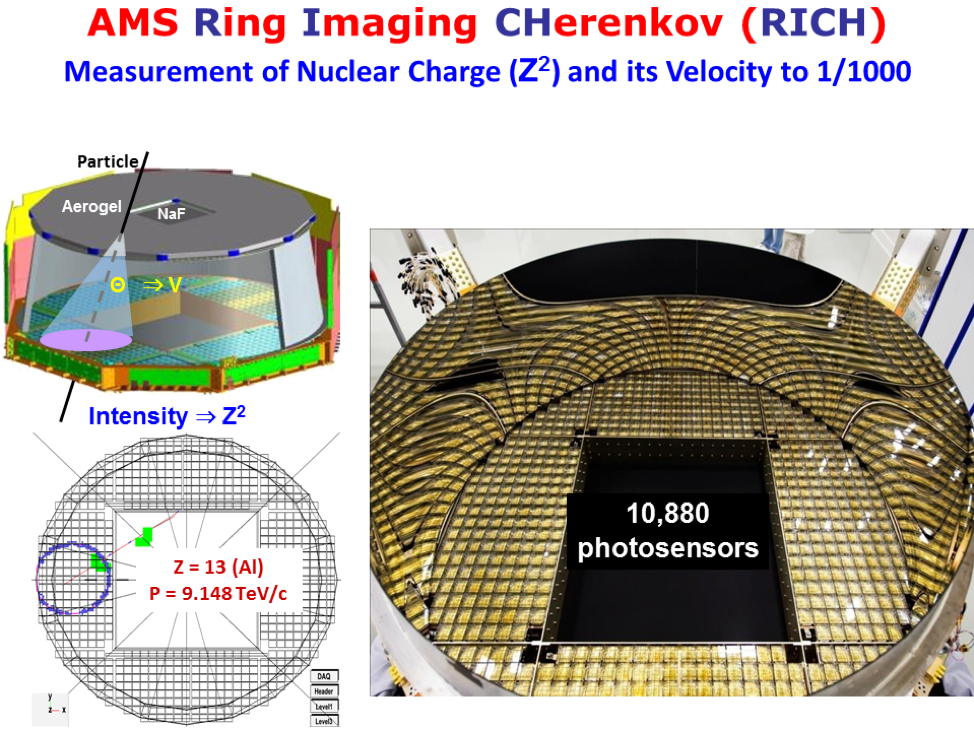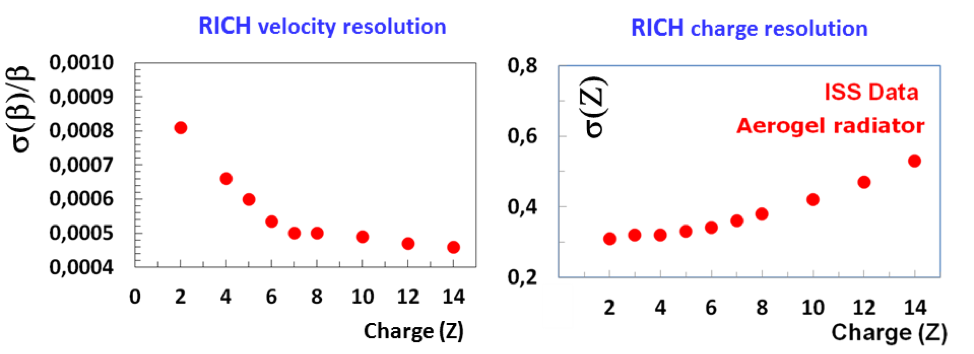# The Ring Imaging Cherenkov Detector (RICH)

The Ring Imaging Cherenkov (RICH) detector measures velocity and $Z$. It consists of two radiators, an expansion volume, and a photodetection plane shown in Figure 1. The dielectric radiators induce the emission of a cone of Cherenkov photons when traversed by charged particles with a velocity greater than the velocity of light in the material. The central radiator is formed by 16 sodium fluoride, NaF, tiles, each 85 x 85 x 5 mm3, with a refractive index $n=1.33$. These are surrounded by 92 tiles, each 115 x 115 x 25 mm3, of silica aerogel with a refractive index $n=1.05$. This allows the detection of particles with velocities $\beta >0.75$ for those which pass through the NaF radiator and $\beta > 0.953$ for those which pass through the aerogel radiator.  The expansion volume extends along z for 470 mm below the radiators and above the photodetection plane.  It is surrounded by a high reflectivity mirror to increase detection efficiency.  The photodetection plane is an array of 10,880 photosensors in multi-channel photomultiplier tubes with an effective spatial granularity of 8.5 x 8.5 mm2. The opening angle of the Cherenkov radiation cone is a measure of the velocity of the incoming charged particle. Typical velocity resolution is $\sigma(\beta)/\beta = 0.1%$ for $|Z|=1$ particles traversing the aerogel.  The sum of the signal amplitudes is proportional to $Z^2$ resulting in a typical charge resolution of $\sigma(Z)=0.32$ for $Z=6$. Figure 2 shows the velocity and charge resolution versus $Z$.Figure 1.  The RICH detector and an event display with $Z=13$ and $P = 9.148$ TeV/c.Figure 2. The RICH velocity and charge resolution vs charge $Z$.